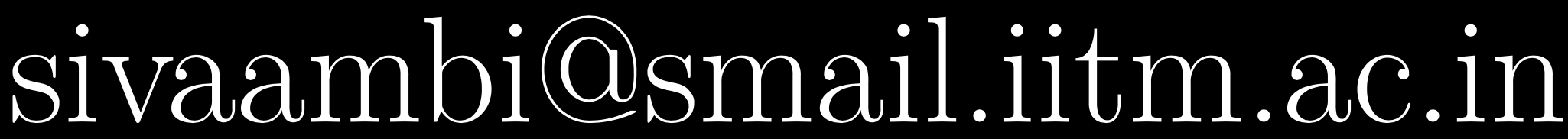# Probability, Statistics and Stochastic Processes, Jul-Nov 2021

### Instructor

Sivaram Ambikasaran,### Syllabus

Probability Axiomatic definition of probability, Independent Events, Baye's theorem, Discrete and continuous random variables, Distribution, Functions of random variables, Expectation, Variance, Correlation coefficient, Chebyshev’s inequality, Markov inequality, Conditional expectations, Standard discrete and continuous distributions (Binomial, Poisson, Geometric, Exponential, Normal, Chi-square), Moment generating function, Sums of random variables, Law of large numbers, Central limit theorem, Normal approximation to Binomial.
Statistics Sampling distribution, Estimation of parameters, Maximum likelihood estimates, Confidence intervals, Student’s t-distribution, Testing hypothesis, Goodness of fit.
Stochastic Processes Discrete random process, Stationary random process, Bernoulli process, Poisson process, Markov chain.

### Textbooks

• Introduction to Probability, by Dimitri Bertsekas and John N. Tsitsiklis (referred as BT in online lectures)

### Other Reference Textbooks

• Introductory Probability and Statistical Applications, by Paul L. Meyer (referred as PLM in online lectures)
• John E. Freund's Mathematical Statistics with Applications, by Irwin Miller and Marylees Miller (referred as M$$^2$$ in online lectures)
• Fundamentals of Applied Probability and Random Processes, by Oliver C. Ibe (referred as Ibe in online lectures)